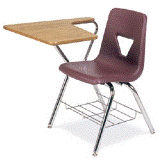## Mathematics, Department of## Math Department: Class Notes and Learning Materials

2010

#### Abstract

Topics include: Semicontinuity, equicontinuity, absolute continuity, metric spaces, compact spaces, Ascoli’s theorem, Stone Weierstrass theorem, Borel and Lebesque measures, measurable functions, Lebesque integration, convergence theorems, Lp spaces, general measure and integration theory, Radon- Nikodyn theorem, Fubini theorem, Lebesque-Stieltjes integration, Semicontinuity, equicontinuity, absolute continuity, metric spaces, compact spaces, Ascoli’s theorem, Stone Weierstrass theorem, Borel and Lebesque measures, measurable functions, Lebesque integration, convergence theorems, Lp spaces, general measure and integration theory, Radon-Nikodyn theorem, Fubini theorem, Lebesque-Stieltjes integration.

COinS